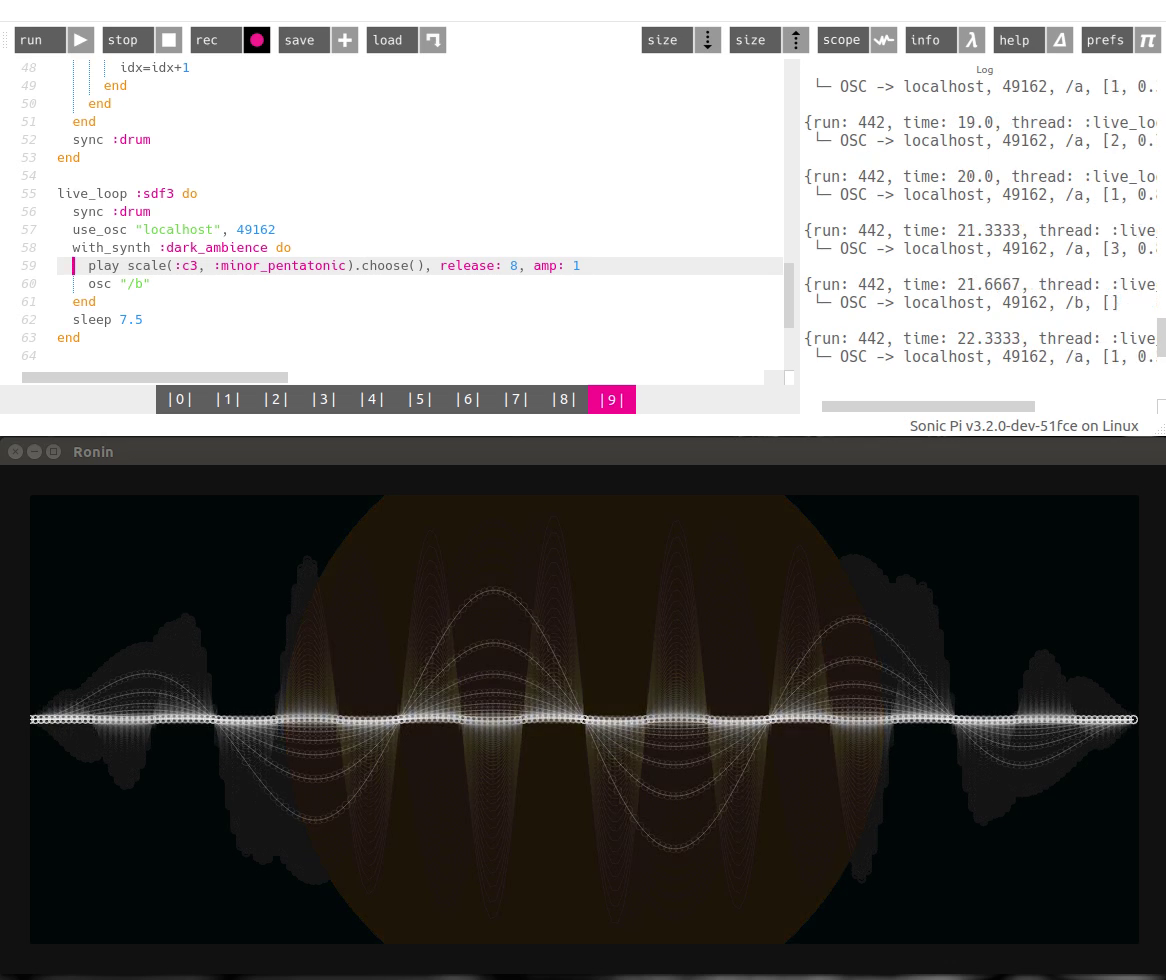## ronin experiment 19 - Sonic Pi visualizer

I created a short sonic pi session and wrote a visualizer for it in ronin. Sonic pi sends osc messages that change the amplitude, frequency and decay time of the sine wave based on the bass sound and triggers random colored circles when the pad sound is played# This is the sonic pi code for the session

``````bd = "bd/bd_013.wav"
sn = "sn/sn_053.wav"
hh = "hh/hh_061.wav"
oh = "hh/hh_062.wav"

d_bd = [3,0,0,0,1,0,0,0,1,0,0,0,1,0,1,0].ring()
d_sn = [0,0,0,0,0,0,0,1].ring()
d_hh = [1,1,0,0].ring()
d_oh = [0,0,1,0].ring()

use_bpm 90

live_loop :drum do
with_fx :echo, mix: 0.04, phase: 0.25 do
t = tick()
if d_bd[t] == 1 then
sample bd
end
if d_sn[t] == 1 then
sample sn
end
if d_hh[t] == 1 then
sample hh, decay: 0.03, decay_level: 0, sustain_level:0
end
if d_oh[t] == 1 then
sample oh, decay: 0.1, decay_level: 0, sustain_level: 00
end
end
sleep 0.5
end
idx = 0
pattern = [1,1,2,1,3].ring()
live_loop :test do
t = tick()

with_fx :echo, mix: 0.3, phase: 0.25 do
with_synth :dpulse do
with_fx :lpf, cutoff: 40 do
n = pattern[idx]
l = rrand(0.3, 0.9)
play scale(:c2, :minor_pentatonic)[n-1], decay: l, decay_level:0, sustain_level:0
play scale(:c4, :minor_pentatonic)[n-1], decay: l, decay_level:0, sustain_level:0
use_osc "localhost", 49162
osc "/a", n, l
end
idx=idx+1
end
end
end
sync :drum
end

live_loop :sdf3 do
sync :drum
use_osc "localhost", 49162
with_synth :dark_ambience do
play scale(:c3, :minor_pentatonic).choose(), release: 8, amp: 1
osc "/b"
end
sleep 7.5
end
``````

# The ronin visualizer looks like this

``````(def d 0.02)
(def state {:amp 1 :next (add (time) 1000) :freq 8 :damp 0.875 })
(defn f (p amp) (
(def h (mul (sin (mul p PI state:freq)) (sub 1 (sq p)) amp ))
(def x (mul p (frame:c)))
(def y (mul h (frame:m)))
{ :x x :y y }
))

(on "/a" (λ (msg) (
(echo (of msg 0))
(set state "amp" 1)
(set state "damp" (sqrt (of msg 1)))
(set state "freq" (mul (of msg 0) 3))
))

(on "/b" (λ (msg) (

(fill
(circle 0 0 300)
(hsl (random 0 365) 100 50 0.3))
)))

(on "animate" '(
(transform:reset)

(fill frame-rect
"#00000005")
(transform:move frame:c frame:m)

(set state "amp" (mul state:amp state:damp))

(map (range -1 1 d) (lambda (l) (
(def p (last (f l state:amp)))
(def q (last (f (sub l d) state:amp)))

(stroke
(circle p:x p:y 4)

(color 255 255 255 0.1) 1)
(stroke
(line  q:x q:y p:x p:y)
(color 255 255 255 0.3) 1))
)))
))
``````

Leave a response

1. Thanks for the Ronin Visualizer. Just came across it when I looked you up following your recent major contribution to Sonic Pi, which I've just compiled. I look forward to trying it out.

btwI have one problem with the Sonic Pi refactoring: the transparency slider on my Mac now no longer has any effect.

2. Would you mind to explain how ronin has to be setup to receive OCS messages?